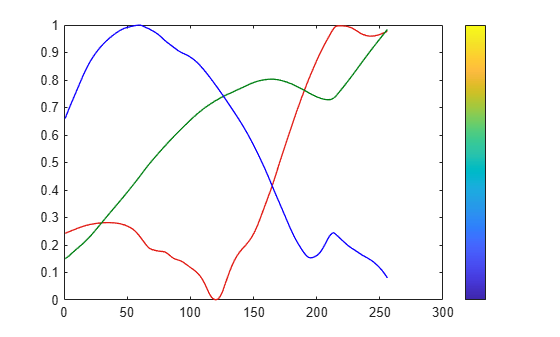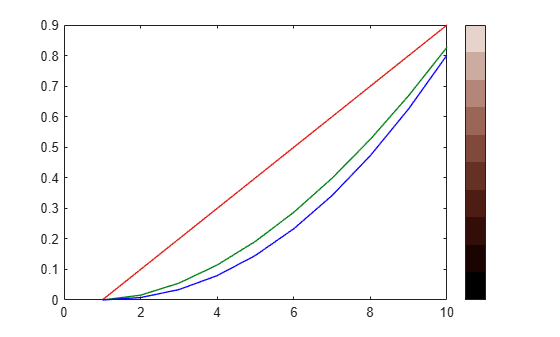# rgbplot

## Syntax

``rgbplot(map)``

## Description

example

````rgbplot(map)` plots the red, green, and blue intensities of the specified colormap.```

## Examples

collapse all

Plot the `parula` colormap, and display a colorbar as a visual reference.

```rgbplot(parula) hold on colormap(parula) colorbar('Ticks',[])```Create a custom colormap and plot it. Then display a colorbar as a visual reference.

```r = (0:.1:.9)'; g = r.^1.8; b = r.^2.1; mymap = [r g b]; rgbplot(mymap) hold on colormap(mymap) colorbar('Ticks',[])```## Input Arguments

collapse all

Colormap to plot, specified as a three-column matrix of RGB triplets. An RGB triplet is a three-element row vector whose elements specify the intensities of the red, green, and blue components of a color. The intensities must be in the range [0, 1]. For example, here is a colormap that contains five colors:

```map = [0.2 0.1 0.5 0.1 0.5 0.8 0.2 0.7 0.6 0.8 0.7 0.3 0.9 1 0];```

This table lists the RGB triplet values for common colors.

ColorRGB Triplet
yellow`[1 1 0]`
magenta`[1 0 1]`
cyan`[0 1 1]`
red`[1 0 0]`
green`[0 1 0]`
blue`[0 0 1]`
white`[1 1 1]`
black`[0 0 0]`

Alternatively, you can create the matrix by calling one of the predefined colormap functions. Call the function as the input argument to the `rgbplot` function. For example, this command plots the `parula` colormap.

`rgbplot(parula)`

Data Types: `double` | `single`